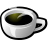NEWS

New version of MANLAB : MANLAB v4.1.7

Contact : guillot@lma.cnrs-mrs.fr

References :

08/31/2019# What is ManLab?

It’s a Matlab package for interactive continuation and bifurcation analysis of non linear systems of equations of the form R(U)=0, where R is a vector of n smooth equations and U a vector of n+1 unknowns.

Manlab provides stability and bifurcation analysis for equilibrium points (fixed points) of dynamical systems.

Manlab also provides algorithms for continuation, stability and bifurcation analysis of periodic orbits of a given dynamical system, using the Harmonic Balance Method.

# The continuation principle in ManLab

The continuation is based on the MAN algorithm  which consists in expanding the unknown U as a formal power series of a path parameter. By using a high order approximation, an accurate and continuous description of the solution branches is obtained.

# Linear stability analysis and Bifurcation analysis

The method of computation of the stability in ManLab is based on the computation of the Floquet exponents in the frequency domain with a Hill eigenvalue problem. Since this problem is multivalued, a special procedure for sorting the most converged Floquet exponents is used. The bifurcations position and type are detected by observing the Floquet exponents crossing the imaginary axis.

See the references  and  for more details about Hill’s method.

# What is the advantage ?

Because the series contains a great deal of information, the control of the continuation and the detection of bifurcation is much easier than with classical predictor-corrector algorithms.

# What is difficult in ManLab ?

For an easy computation of the series in Manlab, the system of equation R(U)=0 must be first written under a polynomial form with a quadratic non-linearity. This is usually the most difficult task for a beginner.

# Programming languages

Manlab is written in the Matlab language, using an object-oriented approach. A graphical interface permits to control the continuation and to analyse the results interactively.

For enhancing the continuation of periodic orbits using HBM (which leads to very large algebraic systems) and for the Hill stability analysis, a Fortran acceleration is also provided.

# Availability

The package is freely available for scientific use. ManLab is a typical research program which is provided "as is", with no guarantee whatsoever.